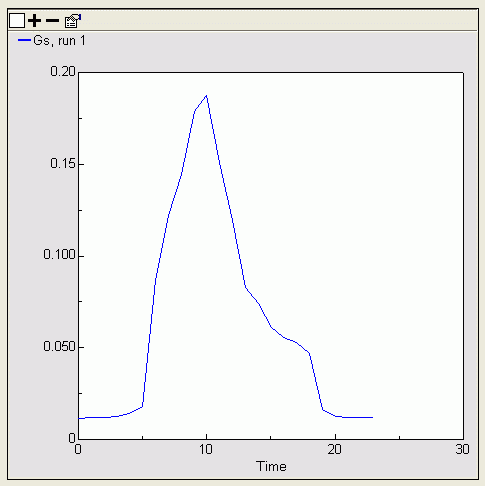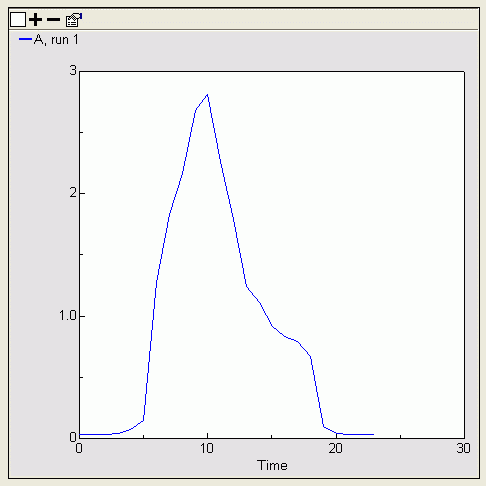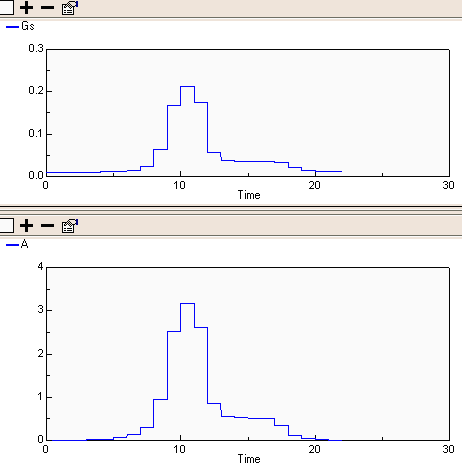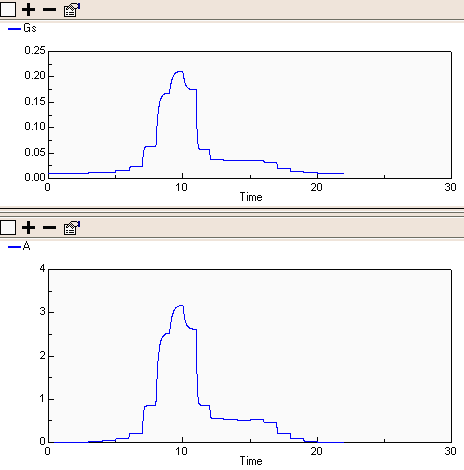# Ecophysiology

## Biome model

ModelId:
biome1
SimileVersion:
5.9

This is closely based on the Prentice and ... Biome model, which aims to model the response of vegetation dynamics to climate change under conditions of water strees. The vegetation does not include any state variables (compartments). This is because the response is assessed in terms of net primary productivity (Pnet), rather than in terms of the consequent accumulation of biomass.

Equations:

```Equations in Desktop
variable: D = graph(month)
variable: month = fmod(time(1),12)
variable: bare = 1-sum([f])
variable: total_transp1 = sum([transp1])
variable: total_transp2 = sum([transp2])

Equations in VEGETATION TYPE
variable: Aa = ap*phi_d*phi_t
Units must be mol(CO2) per month
variable: temp = graph(month)
variable: a = 0.08
"Ratio of leaf respiration to photosynthetic capacity"
(but also (p.653)    described as merely "an empirical parameter")
variable: sigma = (1-alpha/td)^0.5
"sigma is a dimensionless factor that depends only on the
fractional daylength (td) and the ratio of leaf respiration to
photosynthetic capacity (alpha)"
variable: Ap = fpar*sigma*phi*im
"Ap  =  monthly potential photosynthesis"
Units must be mol(CO2) per month
variable: FPAR = 0.5
Value???   "fraction of incoming PAR absorbed by the green vegetation"
variable: Im = graph(month)
Value?????   "total monthly incident PAR"
Units nust be mol(photons) per month
variable: td = 0.5
"fractional daylength"
variable: phi = (if temp<13 then 0.07 else(if temp>38 then 0.04 else 0.04+0.03*(temp-13)/(38-13)))
"quantum efficiency of gross photosynthesis at prescribed ambient CO2"
"mol(CO2)/mol(photons)"
"C3 plants at 13C  =  0.07, at 38C  =  0.04"
"C4 plants  =  0.054, at any temp"
"Field values c. 50% lower"
variable: PHI D = e/d
"drought scalar, ratio of actual to equilibrium evapotranspiration
for the month as calculated by the water flux model"
variable: PHI T = (if temp<5 then 0 else(if temp>35 then 0 else(if temp<20 then(temp-5)/(20-5)else(if temp>30 then(temp-30)/(35-30)else 1))))
"monthly temperature scalar, is set to unity across a range of
temperatures from T2 to T3.   Below T2 the scalar decreases linearly
to a value of zero at a temperature T1, and above T3 the scalar decreases
to zero at a temperature T4"
variable: alpha = z*w1/(z*w1+(1-z)*w2)
variable: f = element([1,0,0,0],index(1))
variable: E = min(s,d)
Instantaneous evapotranspiration rate, mm/hour.   In the paper,
this is "integrated analytically over the 24 hour period".  In the
present model, it is NOT.  Need to think about this:  can we do
numerically, by integrating over the fractiosn of a "typical day"
for each month? (i.e. time unit  =  1 month, time step  =  0.05 'day')
variable: transp1 = alpha*f*e
variable: beta = (1-z)*w2/(z*w1+(1-z)*w2)
variable: transp2 = beta*f*e
variable: Z = element([0.33,0.33,0.9,0.9],index(1))
variable: Wr = z*w1+(1-z)*w2
variable: C = 1
mm/hour   "maximum possible rate of evapotranspiration by plant species"
Value is given in paper.
variable: S = c*wr
"supply function" for plant species.  mm/hour
variable: Pnet = aa-0.4*ap

Equations in WATER
compartment: Water = 100
mm
flow: rain = 40
mm/month
flow: transpiration = transp+transp2
flow: evaporation = 20*bare
variable: W1 = water*thetamax1*d1/(d1+d2)
variable: W2 = water*thetamax2*d2/(d1+d2)
variable: d1 = 500
mm:  depth of upper layer
variable: d2 = 1500
mm:  depth of lower layer
variable: thetamax2 = 200
variable: thetamax1 = 300
```

## Iterative solution to Ball-Berry stomatal conductance simultaneous equations (Simile V4+)

ModelId:
BallBerry4a
SimileVersion:
5.9

The Ball-Berry stomatal conductance poses a problem for conventional System Dynamics modelling tools because it depends upon the solution of the following pair of simultaneous equations.

Gs = g0 + g1 * A * H / Ca
A = Gs * AQ

Equations:

Equations in BallBerry4aP

Equations in Environment

Variable C_a : Carbon dioxide concentration (umol CO2 (mol air)^-1)
C_a = graph(time())
Typical diurnal curve in forest canopy

Variable H : Relative humidity (proportion)
H = graph(time())
Typical diurnal graph (24 hour)

Variable Q : Photon flux density (umol m^-2 s^-1)
Q = graph(time())
Graph for a sunny day (24 hours)

Equations in Ball-Berry

Variable Gs_start
Gs_start = if time()==0 then g_0 else last(Gs_0)
Where:
Gs_0=Iteration time step/Gs_0

Variable g_0 : Stomatal conductance in the dark (mol m^-2 s^-1)
g_0 = 0.01

Variable g_1 : Ball-Berry stomatal conductance coefficient
g_1 = 23

Equations in Iteration time step

Alarm
Variable Gs
Gs = if loop_count==0 then Gs_start else Gs_0
Where:
Gs_start=../Gs_start

Variable Gs_0 : Stomatal conductance (mol m^-2 s^-1)
Gs_0 = g_0+g_1*A*H/C_a
Where:
A=Assimilation/A
H=../../Environment/H
C_a=../../Environment/C_a
g_0=../g_0
g_1=../g_1
Ball-Berry equation

Variable loop_count
loop_count = iterations(al1)

Equations in Assimilation

Variable A : Assimation (umol CO2 m^-2 s^-1)
A = A_Q*Gs
Where:
Gs=../Gs

Variable A_Q : Assimilation light response curve
A_Q = graph(Q)
Where:
Q=../../../Environment/Q
Relationship of Assimilation with photon flux density (light) when stomatal conductance (Gs) is maximum

Results:Plot of stomatal conductance versus time            Plot of assimilation versus time

## Iterative solution to Ball-Berry stomatal conductance simultaneous equations (Simile V3+)

ModelId:
ballberry1
SimileVersion:
3.1+

Here’s an example of a model with two simultaneous equations implemented in Simile. It is a version of the Ball-Berry stomatal conductance model where assimilation (photosynthesis) is a function of stomatal conductance and stomatal conductance is a function of assimilation.

Where there is a big change in stomatal conductance (Gs) more iterations are needed to reach a certain accuracy.

Category:
Specific
Equations:

Equations in Desktop

Equations in Assimilation

Variable A : Assimation (umol CO2 m^-2 s^-1)
A = A_Q*last(Gs_0)
Where:
Gs_0=../Gs

Variable A_Q : Assimilation light response curve
A_Q = graph(Q)
Where:
Q=../../../Environment/Q
Relationship of Assimilation with photon flux density (light) when stomatal conductance (Gs) is maximum

Equations in Environment

Variable C_a : Carbon dioxide concentration (umol CO2 (mol air)^-1)
C_a = graph(time())
Typical diurnal curve in forest canopy

Variable H : Relative humidity (proportion)
H = graph(time())
Typical diurnal graph (24 hour)

Variable Q : Photon flux density (umol m^-2 s^-1)
Q = graph(time())
Graph for a sunny day (24 hours)

Equations in Iteration

Variable Gs : Stomatal conductance (mol m^-2 s^-1)
Gs = if time()==time then Gs else g_0+g_1*A*H/C_a
Where:
A=Assimilation/A
time=../time
H=../../Environment/H
C_a=../../Environment/C_a
g_0=../g_0
g_1=../g_1
Gs=../Gs
Ball-Berry equation

Variable errorGs
errorGs = Gs_0-last(Gs_0)
Where:
Gs_0=Gs

Equations in Ball-Berry

Variable A
A = A
Where:
A=Iteration/Assimilation/A

Variable Gs
Gs = if time()==0 then g_0 else last(Gs_0)
Where:
Gs_0=Iteration/Gs

Variable errorGs
errorGs = last(errorGs_0)
Where:
errorGs_0=Iteration/errorGs

Variable g_0 : Stomatal conductance in the dark (mol m^-2 s^-1)
g_0 = 0.01

Variable g_1 : Ball-Berry stomatal conductance coefficient
g_1 = 23

Variable time
time = time()

Results:

This first pair of graphs shows the calculated Stomatal Conductance and Assimilation on an hour-by-hour basis throughout the day.This second pair shows the internal model representation of the two variables converging on the required hourly value. Note that when the magnitude of changes in the variables is large, as it is around time()==10 units, the convergence takes longer.References:

J. T. Ball, I. E. Woodrow, J. A. Berry. 1987. A model predicting stomatal conductance and its contribution to the control of photosynthesis under different environmental conditions. In: Progress in Photosynthesis Research, ed. J. Biggins. M. Nijhoff Publishers, Dordrecht. Vol. 4, pp 221-224.